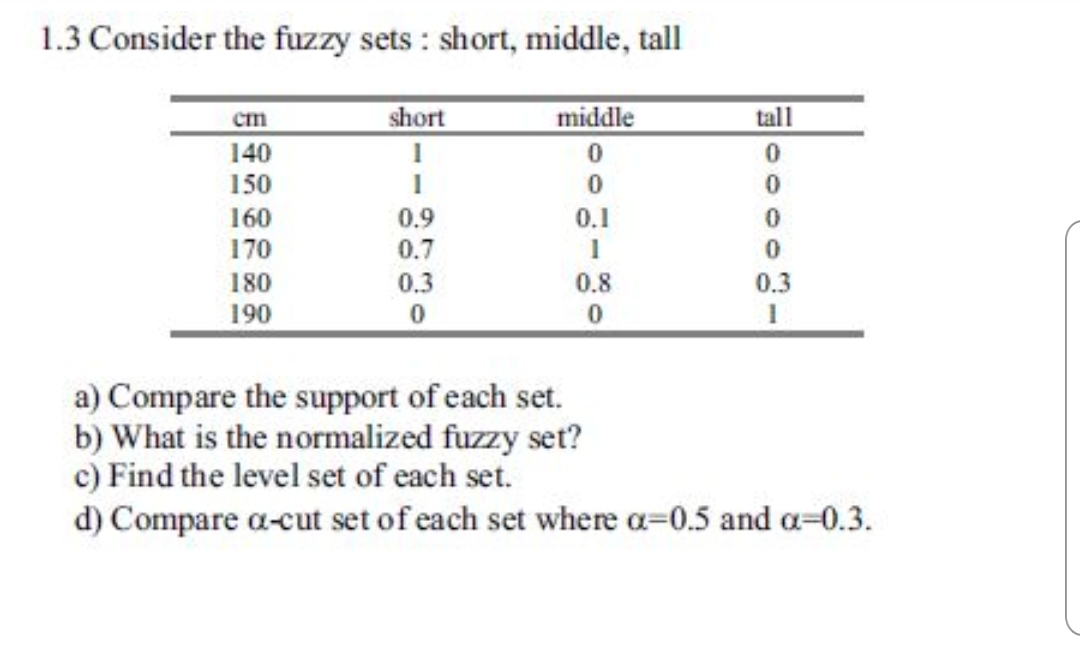# 1.3 Consider the fuzzy sets: short, middle, tallmiddletallshortCIn14015016017000.90.70.30.1000.801801900.30a) Compare the support of each set.b) What is the normalized fuzzy set?c) Find the level set of each set.d) Compare a-cut set of each set where a-0.5 and a-0.3

Question
68 views

the book is "First Course on Fuzzy Theory and Applications"help_outlineImage Transcriptionclose1.3 Consider the fuzzy sets: short, middle, tall middle tall short CIn 140 150 160 170 0 0.9 0.7 0.3 0.1 0 0 0.8 0 180 190 0.3 0 a) Compare the support of each set. b) What is the normalized fuzzy set? c) Find the level set of each set. d) Compare a-cut set of each set where a-0.5 and a-0.3 fullscreen
check_circle

Step 1

As per norms the first three parts a),b) and c) are answered. To determine the various fuzzy properties of the given fuzzy set

Step 2

a) supports of the given fuzzy sets (note that they are all crisp sets)

Step 3

b) A fuzzy set A is normalized if there exists at least one member with maximum membership value, nam...

### Want to see the full answer?

See Solution

#### Want to see this answer and more?

Solutions are written by subject experts who are available 24/7. Questions are typically answered within 1 hour.*

See Solution
*Response times may vary by subject and question.
Tagged in

### Math